# Apps.atoa

•••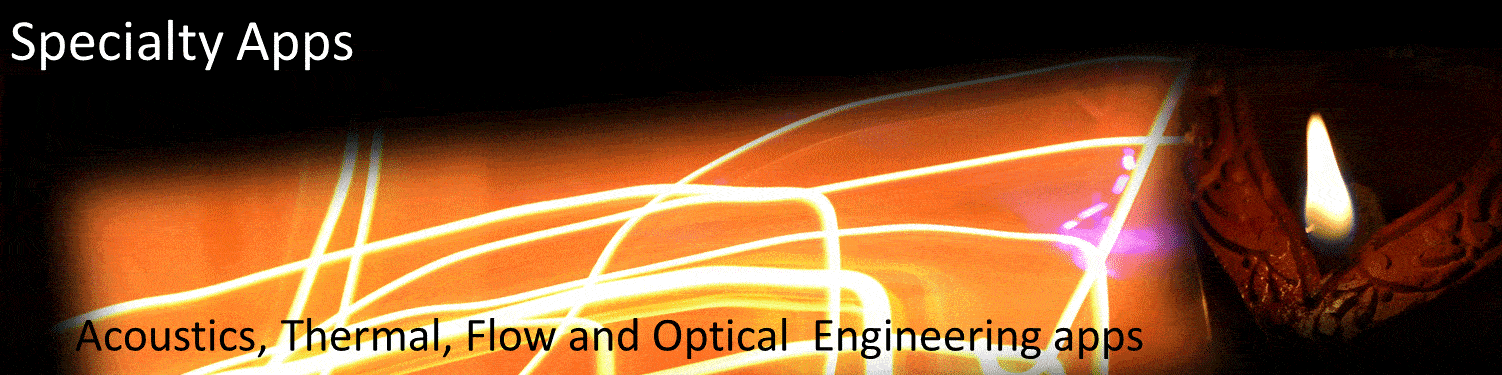•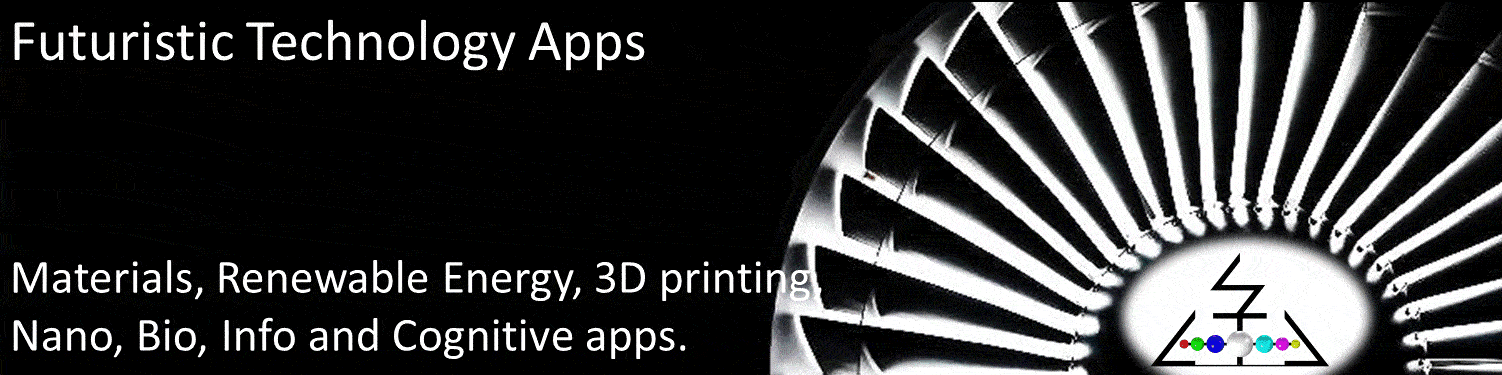•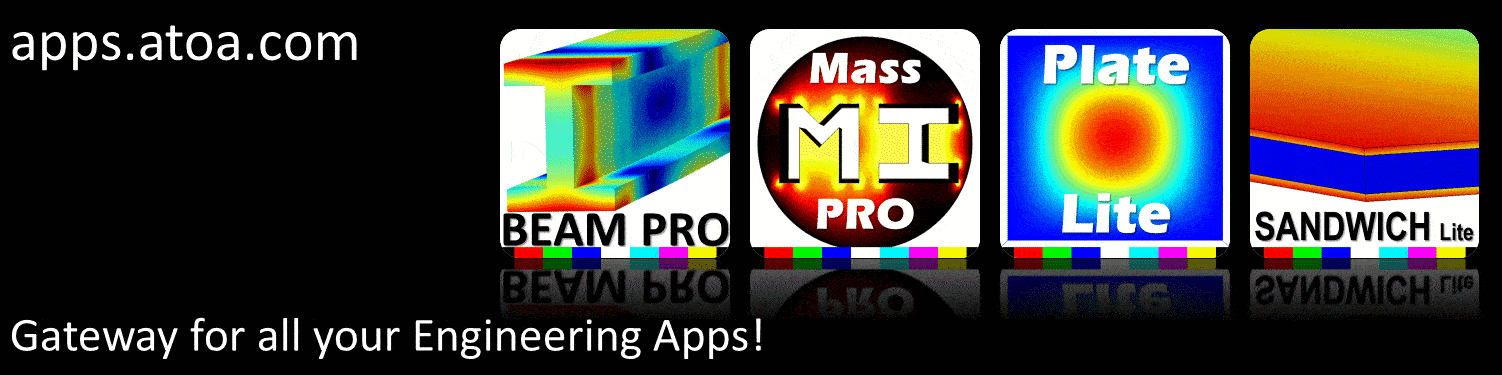•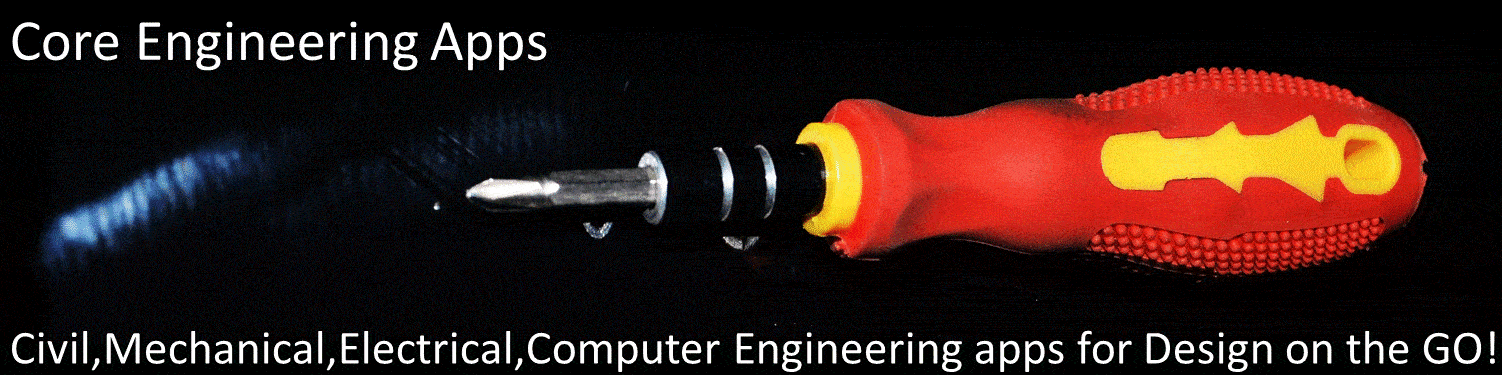### Area moment of Inertia LITE

CALCULATE 2nd MI

Using this tool you can draw any arbitrary shape and calculate Area or 2nd Moment of Inertia and sectional properties of 2D shapes.
These are useful for structural mechanics and dynamics performance prediction.

##### A T O A   A P P S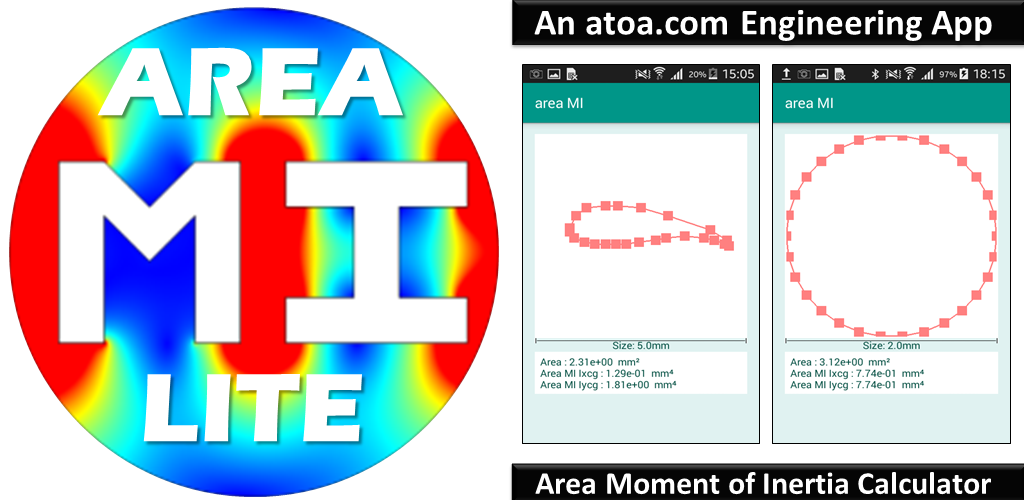##### A P P L I C A T I O N   D E S C R I P T I O N

MI properties for a standard shapes can be obtained from a list of table or standard formula. But for irregular shapes and nonstandard sizes, the sectional properties are not readily available. This tool allows you to draw any shape and then calculate geometric and inertial properties for any arbitrary shape. Geometric and MI properties of any regular/ standard shape can also be predicted.

Draw by tapping the vertex of your 2D shapes. Then click "area MI" to calculate the Sectional properties. The default drawing window size is 2 mm and snap size is 0.02 mm. You can change by tapping the settings. You can also edit the X,Y coordinates of the vertex for more accurate input. Select your convenient Units. Set the window size to the maximum size of your 2D shape. This size can be set to any size from nano meter (10-9) to giga meter (10+9). Area MI lite tool supports any shape bounded outer by straight lines. Draw by tapping the vertex in clock wise or counter clock wise pattern. Avoid crossing of the boundary. The end point can be closed by tapping close end. Clear, Undo, Edit and CloseLoop buttons are useful to start with any shape, edit the vertex to change shapes, add lines, or move lines and or close the boundary.

The second moment of inertia is a measure of the 'efficiency' of any shape to resist bending or flexural loading. Ix and Iy about the centroid are used for flexural or bending resistance prediction. A larger value of the Moment of Inertia indicates that the shapes will show higher the resistance to bend. The polar moment of inertia, Ixy or Jz about centroid, which is the sum of Ix and Iy is used for predicting the torsional resistance of shapes. The centroid location of the area is also important to locate the neutral axis and to calculate other structural properties such as section modulus.

The geometry of any arbitrary shape can be defined using external boundary lines. The area bounded by these lines is calculated using area integration method. The external boundary is represented by linear piece-wise straight lines. A general formula for the moments of arbitrary shape bounded by straight lines is derived leveraging Green's theorem for transforming domain to boundary integrals. This formula is implemented as an algorithm for computing inertial properties.

The tool prediction results for standard shapes are compared with Formulas from text book, Numerical Integration solution using Industrial FEA solvers. For straight lined boundary based geometry the prediction accuracy is very high. For curved boundary the accuracy can be improved by increasing the number of vertex. But for arbitrary shapes such as corroded or damaged members, custom built sections, 3D printed shapes, complex designs, Area MI lite is the only tool which will help to predict sectional properties, cross sectional area and principal Moment of inertia, Ix and Iy.

The number of vertices is limited to 24 in this free version. The pro version will enable you do draw any shape with unlimited vertices (1000+), A, Cx, Cy Ix, Iy, Ixy, Z Properties, Holes, composite sections, data import/export and share functionalities.

##### D O C U M E N T  V I E W E ROur mission is to make " Engineering for all" to "Design on the GO!"